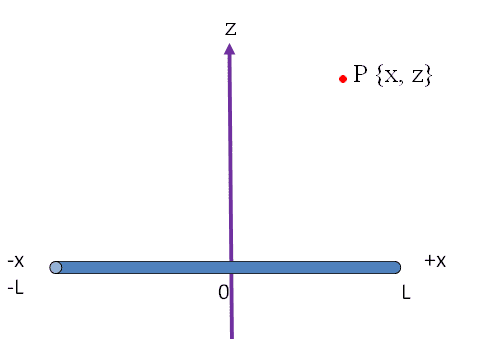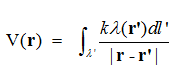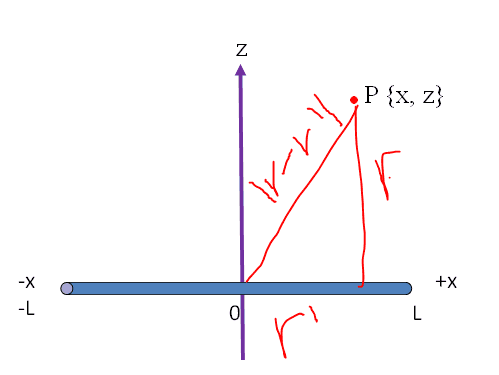# Finding the potential V and electric field E

## Homework Statement

Find the electric potential V and electric field E at the point P due to a line of linear charge density = λ## Homework EquationsE = (2kλ)/z

## The Attempt at a Solution

Now I made these linesIf P is on the z axis, r' = x, but it's shifted, so r' = x-x', and r = z, so then | r - r' | = ((x-x')^2+z^2)^1/2
The dl' = λdx, and -L < x < L
So we use the equation V(r), where k and λ are constants, and integration is from -L to L
So V(z) = kλ ∫ dx / ((x-x')^2+z^2)^1/2 , from -L to L
Am I correct?

And as for the the E field, I think it's simply E = (2kλ)/z ?

## Answers and Replies

blue_leaf77
Science Advisor
Homework Helper
Am I correct?

And as for the the E field, I think it's simply E = (2kλ)/z ?
Your work on the potential is correct. For the field, you need to consider using Gauss law, almost the same as the integral you did for the potential where you need to consider the vector nature of field. The formula for the field you came up with there holds only for infinitely long rod.

rude man
Homework Helper
Gold Member
So V(z) = kλ ∫ dx / ((x-x')^2+z^2)^1/2 , from -L to L
Am I correct?
Almost. What is the variable you're integrating with respect to? and BTW it's V(x,z), not just V(z).

And as for the the E field, I think it's simply E = (2kλ)/z ?
No, it's obviously a function of x and z.
Once you calculated V(x,z) you can get the E field how?

rude man
Homework Helper
Gold Member
Your work on the potential is correct. For the field, you need to consider using Gauss law, almost the same as the integral you did for the potential where you need to consider the vector nature of field. The formula for the field you came up with there holds only for infinitely long rod.
I don't see how Gauss's law applies here.

blue_leaf77
Science Advisor
Homework Helper
OMG, yes I made a reference mistake. I meant Coulomb's law, and the related integral over the rod's length.

Almost. What is the variable you're integrating with respect to? and BTW it's V(x,z), not just V(z).

No, it's obviously a function of x and z.
Once you calculated V(x,z) you can get the E field how?

Integrate with respect to x, since the charges lie on the x axis

For the E field, I believe I should use dE = (kλdx z) / r^3 , and in our case the r = ((x-x')^2+z^2)^1/2
Or E = -del V

Last edited:
rude man
Homework Helper
Gold Member
Integrate with respect to x, since the charges lie on the x axis
The charges are on the x' axis. Your answer had better include x, right? which won't happen if you integrate with respect to x, letting x = +L and -L as limits of integration. This might have been a typo on your part; the answer is the same other than winding up with V(x',z) instead of V(x,z).
For the E field, I believe I should use dE = (kλdx z) / r^3 , and in our case the r = ((x-x')^2+z^2)^1/2
Or E = -del V
E is a vector, so your first expression can't be right.
I do suggest the second one, though ...
Be prepared for some messy math, I suggest going to math software if you have access to it.

The charges are on the x' axis. Your answer had better include x, right? which won't happen if you integrate with respect to x, letting x = +L and -L as limits of integration. This might have been a typo on your part; the answer is the same other than winding up with V(x',z) instead of V(x,z).
E is a vector, so your first expression can't be right.
I do suggest the second one, though ...
Be prepared for some messy math, I suggest going to math software if you have access to it.

Yeah I will use mathematica to solve these. And thanks for clearing it up, I was a little confused on the integral, got it now. :)